Home > Mini Projects > 2 Bit Parallel or Flash Analog to Digital Converter

2-Bit Parallel or Flash Analog to Digital Converter

Abstract -A 2 bit parallel analog to digital converter using comparators and priority encoder giving digital output is proposed, designed, and implemented using transistor-transistor logic . The circuit is found to be working satisfactorily . this is also called as flash ADC.

# I. Introduction

» An analog-to-digital converter (A/D or ADC) produces a digital number corresponding to an analog input voltage sample.

» Flash ADC Consists of a series of comparators, each one comparing the input signal to a unique reference voltage.

» The comparator outputs connect to the inputs of a priority encoder circuit, which produces a binary output

# II. Design and Working

Input voltage 0-5v. Output -2 bit binary. Any input voltage between 0-5v will be converted into corresponding binary output.

The circuit shown in figure takes the given analog signal as input (varying from 0-5v) and produces a digital output corresponding to the input given. In this circuit ,we used three comparators and a 4*2 priority encoder. There are four resistors of equal value of 1kohm each. And the reference voltage is taken as 5volts.

A. According to voltage division rule, the reference voltage given is divided into 3Vr/4 ,2Vr/4, Vr/4 at posituve terminal of the comparators respectively. Here, 'Vr' is the reference voltage. Span is defined as the difference between maximum and minimum analog input voltages and here, it is 5v. step size is given by span/2^n where n is number of bits in the binary output(here, n=2). Hence, stepsize is 5/4=1.25 (here).

B. Now, the negative terminals of the comparators are given the input voltage as analog signal and by comaparing the positive and negative terminals, as the analog input voltage exceeds the reference voltage at each comparator, the comparator outputs will go to a high state. By considering first comparator, the negative terminal has input voltage 'Va' and the positive terminal has input as '3Vr/4' . if Va is greater than 3Vr/4, the output will be logic 1; else the output will be logic 0. Similarly, the same procedure is repeated for the remaining comparators with their respective reference voltages.

C. The outputs of comparators are given as inputs to the priority encoder and the corresponding binary outputs are generated. The priority encoder generates a binary number based on the highest-order active input, ignoring all other active inputs. The output of the first comparator will be higher priority input compared to other bits.

Simplest of all analog to digital converters. Most efficient in terms of speed, very fast; limited only in terms of comparator and gate propagation delays.

# IV. INPUT RANGES AND CORRESPONDING OUTPUT

 Input values Output 0-1.25 00 1.25-2.5 01 2.5-3.75 10 3.75-5 11

# V. CIRCUIT DIAGRAM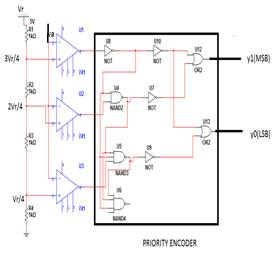Fig 1

# VI. Figures and Results

#### The output of priority encoder corresponding to different inputs :

A. Input=5v, output=11

####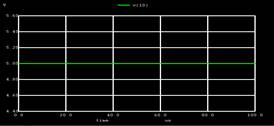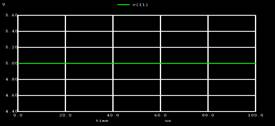B. Input=3v, output =10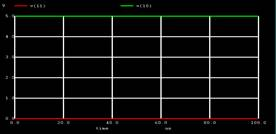C. Input=2v, output=01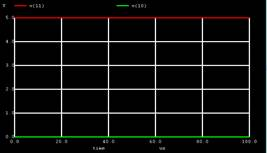D. Input=1v, output=00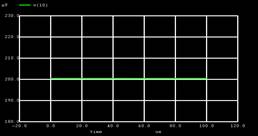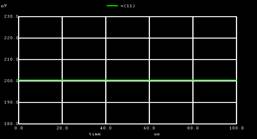# VII. CODES

*analog to digital

.include opamp.cir

.include spe.cir

r1 1 2 1k

r2 2 3 1k

r3 3 4 1k

r4 4 0 1k

x1 5 2 6 opamp

x2 5 3 7 opamp

x3 5 4 8 opamp

x4 6 7 8 9 10 11 prior

vr 1 0 dc 5

va 5 0 dc 1

v1 9 0 dc 5

.tran 1u 100us

.control

run

display

plot v(10)

plot v(11)

set xbrushwidth=3

.endc

.end

SUBCIRCUITS :

*priority encoder

.subckt prior 1 3 5 7 11 12

.include snot.cir

.include ors.cir

.include nand.cir

.include subnand3.cir

x1 1 2 not

x2 2 3 4 nand1

x3 4 2 5 6 nand3

x4 4 9 not

x5 6 10 not

x6 1 9 11 or

x7 1 10 12 or

.ends prior

*opamp

.subckt opamp 1 2 6

r1 1 2 10meg

e1 3 0 1 2 100k

r2 3 4 1k

c1 4 0 15u

e2 5 0 4 0 1

r3 5 6 10

.ends opamp

*not

.subckt not 1 3

.model mosfet1 pmos

.model mosfet2 nmos

m1 3 1 2 2 mosfet1

m2 3 1 0 0 mosfet2

vdd 2 0 dc 5

.ends not

*2 input nand

.subckt nand1 2 3 4

.model mos1 pmos

.model mos2 nmos

m1 4 2 1 1 mos1

m2 4 3 1 1 mos1

m3 4 2 5 0 mos2

m4 5 3 0 0 mos2

v1 1 0 dc 5

.ends nand1

*3 input nand

.subckt nand3 1 2 3 6

.include nand.cir

x1 1 2 4 nand1

x2 4 4 5 nand1

x3 5 3 6 nand1

.ends nand3

*or gate

.subckt or 1 3 5

.include nand.cir

x1 1 1 2 nand1

x2 3 3 4 nand1

x3 2 4 5 nand1

.ends or